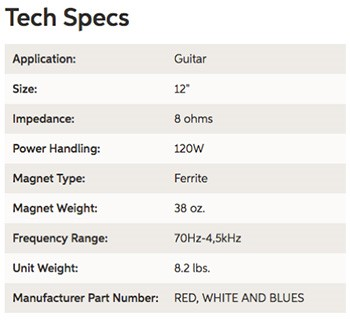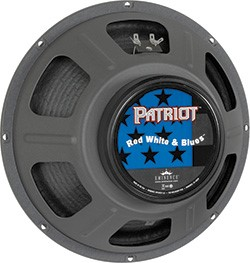Currently viewing Vol. 6 • Issue 6 • 2019# Guitar Speakers: Resistance vs. Impedance – What’s the Difference

Used with permission from: https://www.sweetwater.com/insync/guitar-speakers-resistance-vs-impedance-whats-difference/?utm_content=article1-button&utm_source=insync&utm_medium=email&utm_campaign=20190406

Editor’s note: From time to time, questions arise about the correct usage of the term “impedance” versus “resistance”. The big difference is that resistance is independent of frequency (which is why it is used when referring to DC where frequency is indeed 0 Hz, and impedance (which is equal to the square root of resistance squared + reactance squared), is related to frequency. As the frequency is increased, the reactance increases so the impedance also increases. This article from Sweetwater.com looks at the usage of these two words for guitar loudspeakers, but it can also be used more generally in acoustics (and electronics).

Do you understand the difference between resistance and impedance when it comes to guitar speakers? Have you ever wondered why certain speakers sound better on certain amps? Do you know how to calculate the impedance when wiring speakers in series or parallel? (No, we’re not going there.)

First off, we know this topic is a little bit tweaky, but at least we’re leaving out all the math, like Ohm’s law, I = V/R, and Z1 + Z2 + Z3 = Z<sub<sum. What we really want you to understand is that resistance and impedance are not the same thing, even though they’re both measured in ohms (the unit of electrical resistance). Does it matter more to your tone than the guitar you choose or the tubes you like? No, but having a good understanding of these fundamentals will definitely allow you to impress your guitar-playing friends. And more importantly, it might save you from blowing up your amp.

## What Is Resistance?

When voltage is applied to an electrical system, that system will exhibit opposition to current, and that opposition is known as resistance. In the case of a speaker, the resistance is a fixed number expressed in ohms. That value, say 8 ohms, is the measured resistance of the voice coil using direct current (DC). Direct current is always at a frequency of 0 Hz.

## Then What Is Impedance?In the case of alternating current (AC) like audio signals, when voltage is applied to a speaker, the impedance changes, dependent on the frequency applied to it. The number that you see on a spec sheet is “nominal impedance,” which is the “mean” of the speaker’s resistance. So if a speaker’s nominal impedance rating is 8 ohms (like you’ll see in the spec field on our site), in use it may peak as high as 70 ohms or go as low as 2.5 ohms — depending on the frequency applied — but about 8 ohms is where it spends most of its time.

## Here’s an ExampleThe graph below shows two measurements — impedance and frequency response — both plotted against the frequency response (the numbers across the bottom of the chart, from 20Hz to 20kHz) of an Eminence Red White and Blues speaker with an 8-ohm (nominal) rating.

The peach-colored line shows the actual impedance when different frequencies are applied to a speaker (the impedance in ohms, which is displayed by the numbers along the right side of the graph). But if you look at the peach-colored line (impedance), you’ll notice that it only measures 8 ohms at three frequencies: 50Hz, 200Hz, and 500Hz. The red line is the speaker’s frequency response (the loudness in dB SPL, which is displayed by the numbers on the left side of the graph). Notice the sharp peak of almost 70 ohms just above 100Hz followed by a return to 8 ohms, then a steady climb to 50 ohms starting at about 350Hz and ending at 20kHz.

## Why It Matters

Matching a speaker’s impedance rating (nominal) to an amplifier’s impedance rating is critical for optimal performance and safety. However, as you can see from the graph above, the actual demands that a speaker places on an amplifier’s output transformer are quite different than a fixed number. This becomes more evident when more than one speaker is connected to an amplifier, because more variables are introduced. The variables are even greater when you factor in the impedance-handling differences between tube and solid-state power amps.

Putting this in a practical application, let’s take the example of the Dr. Z Z-Master amplifier. This amp uses a 4-ohm output transformer and three 8-ohm speakers wired in parallel. This creates a safe impedance mismatch that actually contributes to the sonic outcome of the amplifier. Here’s what Mike Zaite, Dr. Z himself, has to say about speaker impedance in the real world.

“Speaker impedance is not a fixed number; it’s more nuanced. Speakers are rated in AC impedance, and in use, it varies with frequency. The nominal impedance of the speakers in the Z-Master are 8 ohms per speaker. Using Ohm’s law, the nominal impedance of three 8-ohm speakers wired in parallel is 2.6666…rounded up to 2.67. However, Ohm’s law is used to figure DC resistance, so this number is not indicative of practical use, since the AC impedance of a speaker changes depending upon the frequencies it is reproducing at any given time. Kirchhoff’s laws would be more appropriate for solving this problem. So, taking into account the relative frequency range of the electric guitar in application and calculating impedance based on three speakers in use, the actual speaker parallel load is roughly 3.2 ohms, which represents the average AC load that the speakers reflect on the output transformer. Using a 4-ohm output transformer creates the slight mismatch that is part of the Z-Master sound.”

I hope this shines a light on a subject that can be pretty shadowy. The main takeaway is that speaker impedance is not a static value — and that impedance value has an effect on your power amp and ultimately the sound being produced.